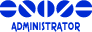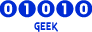## Aldebaran and alde_in loop

Forum closed. All further discussion to be discussed at https://github.com/OpenKore/

Moderators: Moderators, Developers

Message
Author
levantine
NoobPosts: 10
Joined: 12 Jul 2011, 11:37
Noob?: Yes

### Aldebaran and alde_in loop

I just encountered the same problem that i saw on a thread in Jan 2011. When the bot goes into alde_in to sell/buy things, it will be stuck in a loop because upon exiting the shop, it will try to go to the lockmap, but the route to the lockmap will pass the warp into the shop (alde_in), causing a loop.

Calculating lockMap route to: Border Checkpoint(yuno_fild12)
Map Change: aldebaran.gat (197, 68)
Map Change: aldeba_in.gat (94, 41)
Calculating lockMap route to: Border Checkpoint(yuno_fild12)
Map Change: aldebaran.gat (197, 68)
Map Change: aldeba_in.gat (94, 41)

I saw a post with a solution whereby we can edit the aldebaran.fld with a hex editor and making the positions (199, 69)(199, 68)(199, 67) to be un-walkable (01).

I have downloaded the Hex Editor and opened the aldebaran.fld but I do not know how to identify the position stated above. Can anyone help?

returner
NoobPosts: 1
Joined: 28 Feb 2012, 23:31
Noob?: Yes

### Re: Aldebaran and alde_in loop

I wish someone can help with this, problem. I've tried everything but I just can't avoid this loop in alde_in and aldebaran.

levantine
NoobPosts: 10
Joined: 12 Jul 2011, 11:37
Noob?: Yes

### Re: Aldebaran and alde_in loop

I manage to get a reply from the guy who solved this, you need to use a hex editor for this. What we want is to make the positions (199, 69)(199, 68)(199, 67) to be un-walkable (01).

Open the aldebaran.fld

The first 4 bytes is width and height of the map, start from the 5-th byte, each byte for a point.
For example, the aldebaran.fld, the first 4 bytes is 18 01 18 01.
So, the first two bytes '18 01' is the width and the second two bytes '18 01' is height.
Then, the width = 0x0118 = 280, the heith = 0x0118 = 280.
The offset of (199, 69) is 199 + 69 * 280 + 4 = 19523 = 0x4C43.
The offset of (199, 68) is 199 + 68 * 280 + 4 = 19243 = 0x4B2B.
The offset of (199, 67) is 199 + 67 * 280 + 4 = 18963 = 0x4A13.

198 + 69 * 280 + 4 = 19591 = 4C87
198 + 68 * 280 + 4 = 19242 = 4B2A
198 + 67 * 280 + 4 = 18962 = 4A12

kLabMousePosts: 1301
Joined: 24 Apr 2008, 12:02

### Re: Aldebaran and alde_in loop

levantine wrote:I manage to get a reply from the guy who solved this, you need to use a hex editor for this. What we want is to make the positions (199, 69)(199, 68)(199, 67) to be un-walkable (01).

Open the aldebaran.fld

The first 4 bytes is width and height of the map, start from the 5-th byte, each byte for a point.
For example, the aldebaran.fld, the first 4 bytes is 18 01 18 01.
So, the first two bytes '18 01' is the width and the second two bytes '18 01' is height.
Then, the width = 0x0118 = 280, the heith = 0x0118 = 280.
The offset of (199, 69) is 199 + 69 * 280 + 4 = 19523 = 0x4C43.
The offset of (199, 68) is 199 + 68 * 280 + 4 = 19243 = 0x4B2B.
The offset of (199, 67) is 199 + 67 * 280 + 4 = 18963 = 0x4A13.

198 + 69 * 280 + 4 = 19591 = 4C87
198 + 68 * 280 + 4 = 19242 = 4B2A
198 + 67 * 280 + 4 = 18962 = 4A12
Oh yeah. He forgot to say, that you need remove any file named aldebaran.* except aldebaran.fld. So Openkore can rebuild the penalty rote map.EternalHarvest
DevelopersPosts: 1800
Joined: 05 Dec 2008, 05:42
Noob?: Yes

### Re: Aldebaran and alde_in loop

Actually, the route is being calculated just fine (with route_avoidWalls which is default), but the route_step is so big it just skips the whole part with avoiding the nearest wall. Setting route_step to 10 solves the problem with that particular portal. Ideally, corners like these should be marked/detected and always be used as actual movement points.novacrash
NoobPosts: 1
Joined: 27 Jul 2012, 10:31
Noob?: Yes

### Re: Aldebaran and alde_in loop

You can also avoid re-entering the portal with an automacro... Not ideal, but working and easy to do.

automacro aldebaran {
location aldebaran 197 68
timeout 1
call {
do south
}
}